# Transformation of functions

Transformation of functions

Professor John drew the graph of the function $$f_{1}(x)=x^2$$ on the board.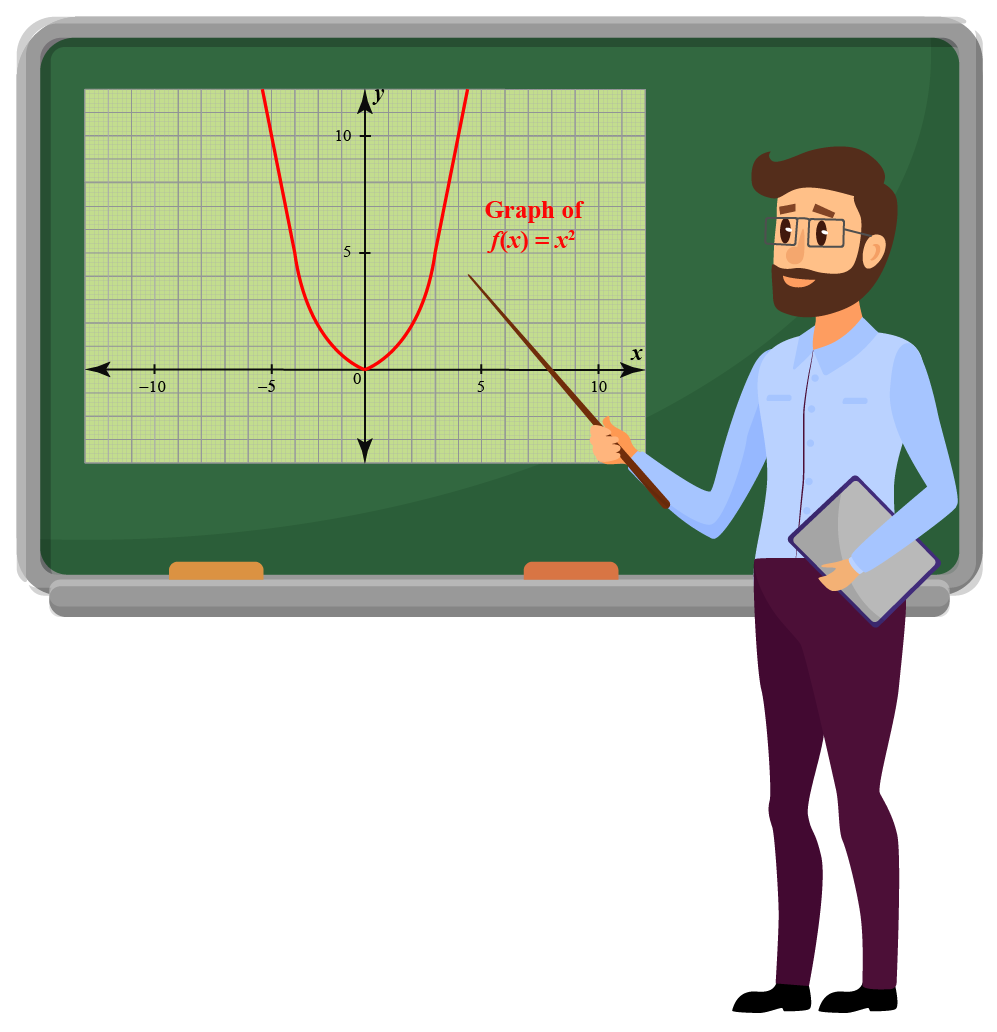The students drew this graph in their note books.

The professor then asked the students to draw the graph of $$f_{2}(x)=x^2+3$$.

Do you think the students would have to draw this graph from scratch?

Do you think there is an easier way to indicate the graph for this function?

Students will not have to create it all over again because $$f_{2}(x)=x^2+3$$ is the function transformation of $$f_{1}(x)=x^2$$.

In this mini-lesson, we will learn about transformations of functions, transformations of exponential functions, function transformation worksheet, and transformations of quadratic functions in the concept of transformation of the functions. Check out the interactive simulations to know more about the lesson and try your hand at solving a few interesting practice questions at the end of the page.

## Lesson Plan

 1 What Is the Meaning of Transformations of Functions? 2 Tips and Tricks on Transformations of Functions 3 Solved Examples on Transformations of Functions 4 Thinking Out of the Box! 5 Interactive Questions on Transformations of Functions

## What Is the Meaning of Transformations of Functions?

The word "transform" means "to change from one form to another."

Transformations of functions mean transforming the function from one form to another.

Transformation of functions is a unique way of changing the formula of a function minimally and playing around with the graph.

Look at the graph of the function $$f(x)=x^2+3$$.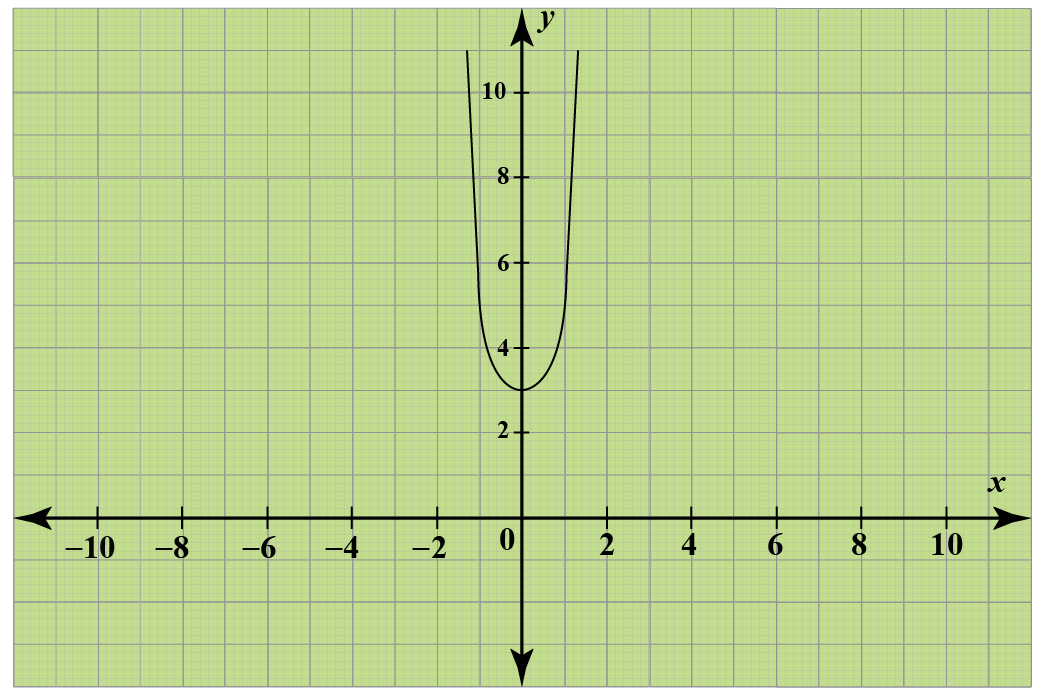Did you observe that the graph is 3 units above the quadratic function $$g(x)=x^2$$?

Visualize this function transformation more clearly by drawing both the functions on the same coordinate plane.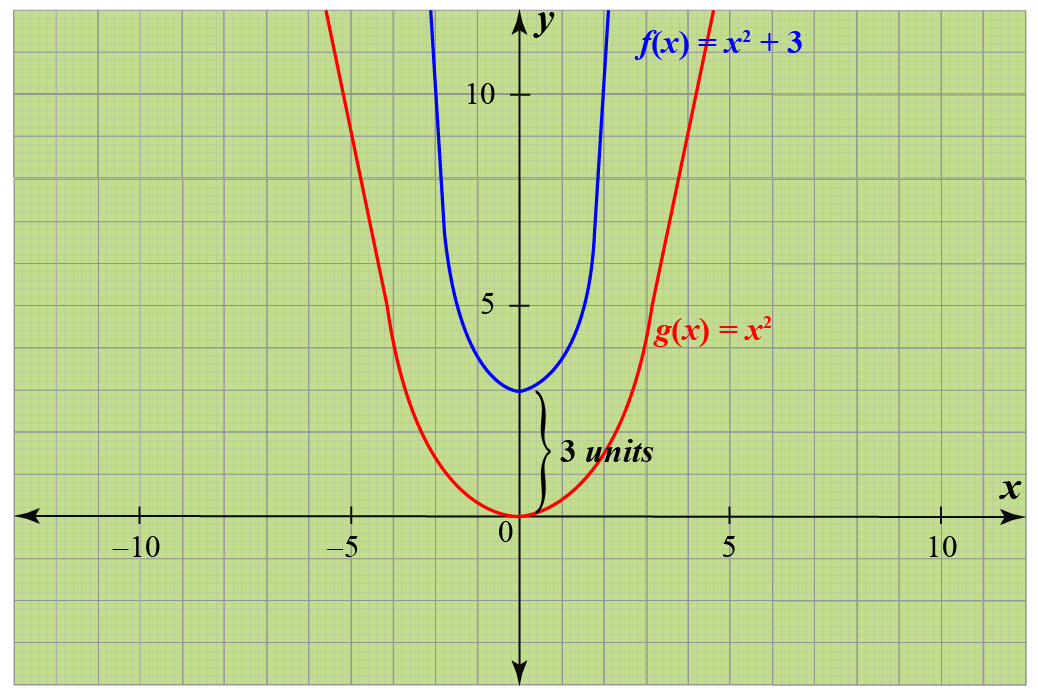## What Are the Types of Transformations of Functions?

The four common types of transformations are translation, rotation, reflection, and dilation.

Transformation Function
Rotation Rotates or turns the curve around an axis.
Reflection Flips the curve and produces the mirror-image.
Translation Slides or moves the curve.
Dilation Stretches or shrinks the curve.

Here is a simulation for you to experiment with all types of transformations.

## How To Make Transformations of a Function?

Here are the rules of transformations of functions that could be applied to the graphs of functions.

We can apply the function transformation rules to graphs of functions.

• Here is the graph of a function that shows the transformation of reflection.

The red curve shows the graph of the function $$f(x) = x^3$$.

The function transformation $$g(x) =- x^3$$ is done and it fetches the reflection of $$f(x)$$ about the $$x$$-axis.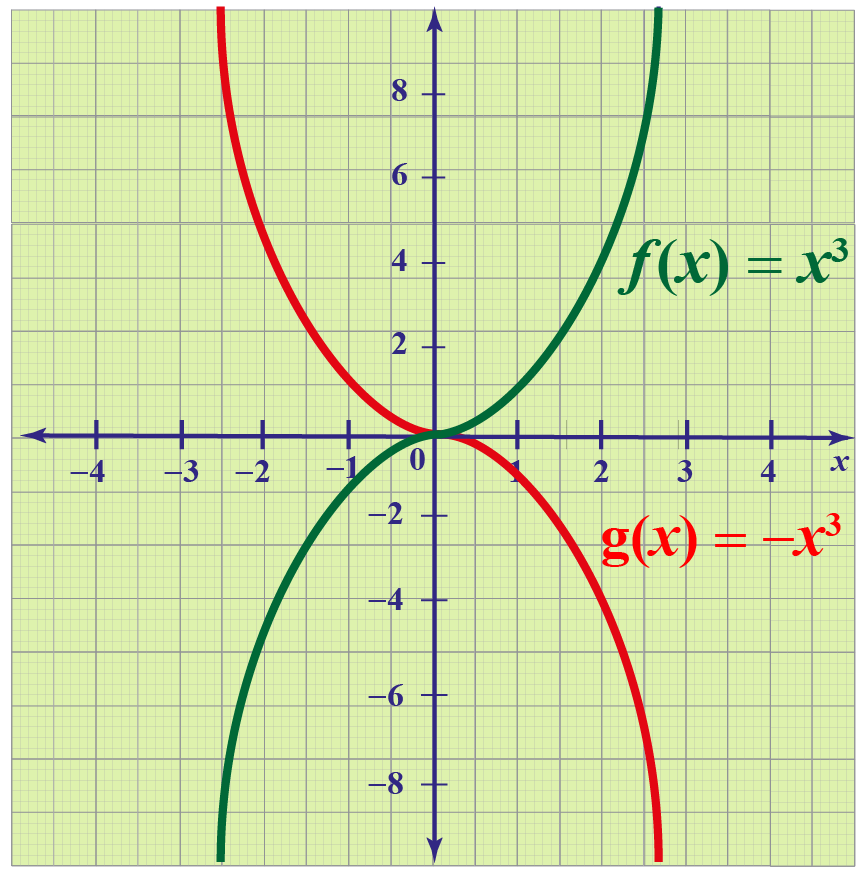• Now, let us observe the transformation of translation.

This red curve shows the graph of the function $$f(x) = x^2$$

The transformation of quadratic function $$f(x) = (x+2)^2$$ shifts the parabola 2 steps right.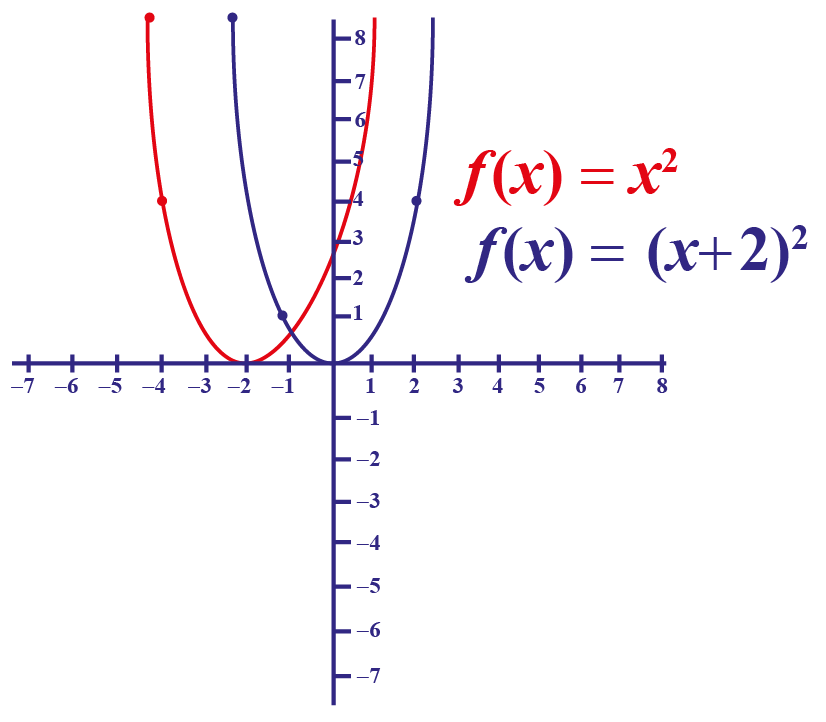• In the function graph below, we observe the transformation of rotation.

To rotate 90$$^\circ$$: $$(x,y)\rightarrow (-y, x)$$

To rotate 180$$^\circ$$: $$(x,y) \rightarrow (-x,-y)$$

To rotate 270$$^\circ$$: $$(x,y) \rightarrow (y, -x)$$

Here we see that the preimage is rotated to 180$$^\circ$$.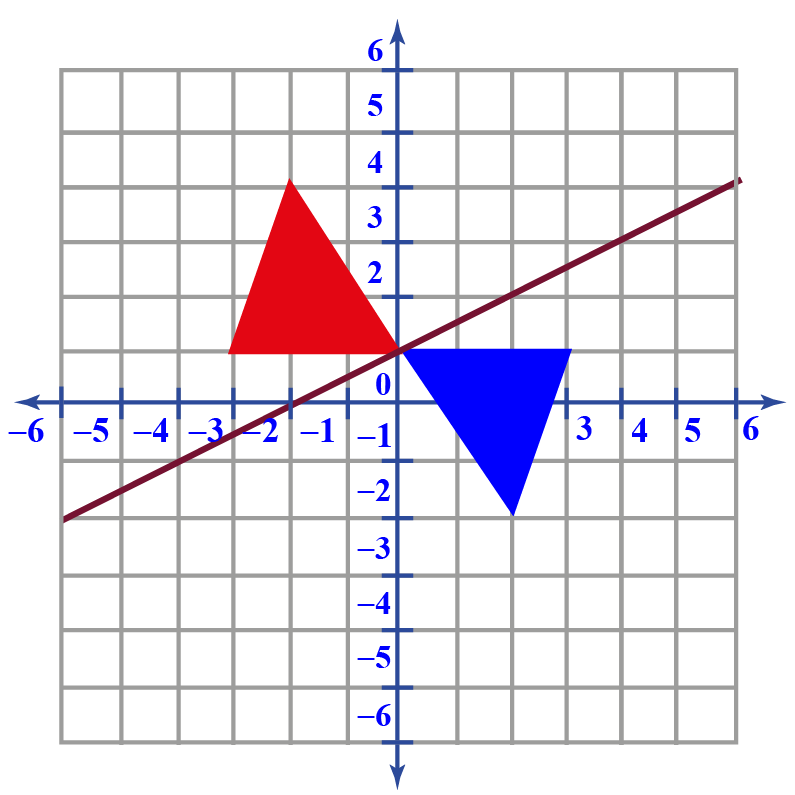Want to know a general way to transform a function?

Here are some tricks for you to transform the given graph of the function.Tips and Tricks
1. $$f(x+c)$$ horizontally shifts the graph of $$f(x)$$ left by $$c$$ units.
2. $$f(x-c)$$ horizontally shifts the graph of $$f(x)$$ right by $$c$$ units.
3. $$f(x)+c$$ vertically shifts the graph of $$f(x)$$ upward by $$c$$ units.
4. $$f(x)-c$$ vertically shifts the graph of $$f(x)$$ downward by $$c$$ units.
5. $$cf(x)$$ vertically stretches the graph of $$f(x)$$ by a factor of $$c$$ units.
6. $$\dfrac{1}{c}f(x)$$ vertically shrinks the graph of $$f(x)$$ by a factor of $$c$$ units.
7. $$f(cx)$$ horizontally shrinks the graph of $$f(x)$$ by a factor of $$c$$ units.
8. $$f\left(\dfrac{x}{c}\right)$$ horizontally stretches the graph of $$f(x)$$ by a factor of $$c$$ units.
9. $$-f(x)$$ reflects the graph of $$f(x)$$ over the $$x$$-axis.
10. $$f(-x)$$ reflects the graph of $$f(x)$$ over the $$y$$-axis.

## Solved Examples

 Example 1

How has the below figure transformed?Solution

The rotation of an image turns its position around an axis.

Thus, here we can observe that the figure is rotated at $$90^{\circ}$$ clockwise.

 Thus, the transformation of rotation can be observed in the figure.
 Example 2

The following graph shows the basic function $$y=b^x$$.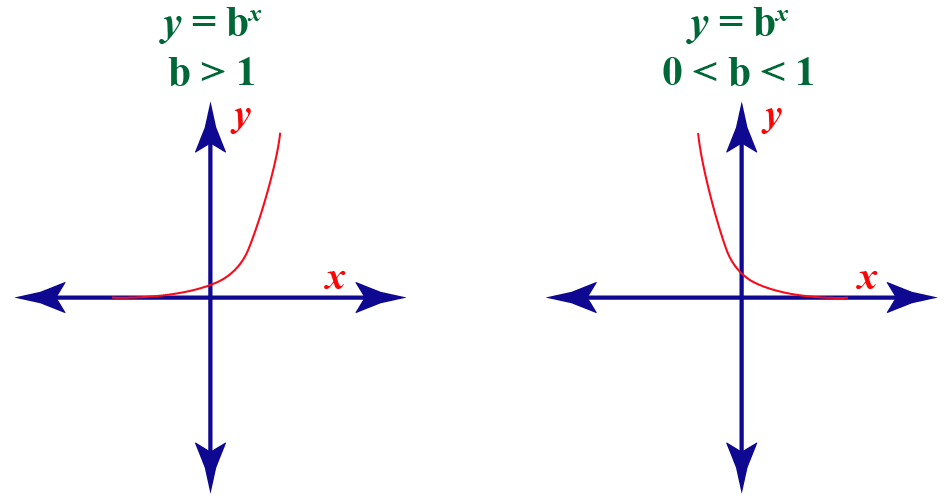Use the function transformation of exponential functions to show the horizontal stretch and the reflection over the $$x$$-axis to draw the graph of the function $$h(x)=-2^{\frac{1}{4}}$$.

Solution

We already know the curve of the basic function $$y=b^x$$, where $$b=2$$.

To stretch the graph horizontally by a factor of 4, we will graph the function $$f(x)=2^{\frac{x}{4}}$$.

To show the reflection over the $$x$$-axis, we will graph the function $$h(x)=-2^{\frac{x}{4}}$$.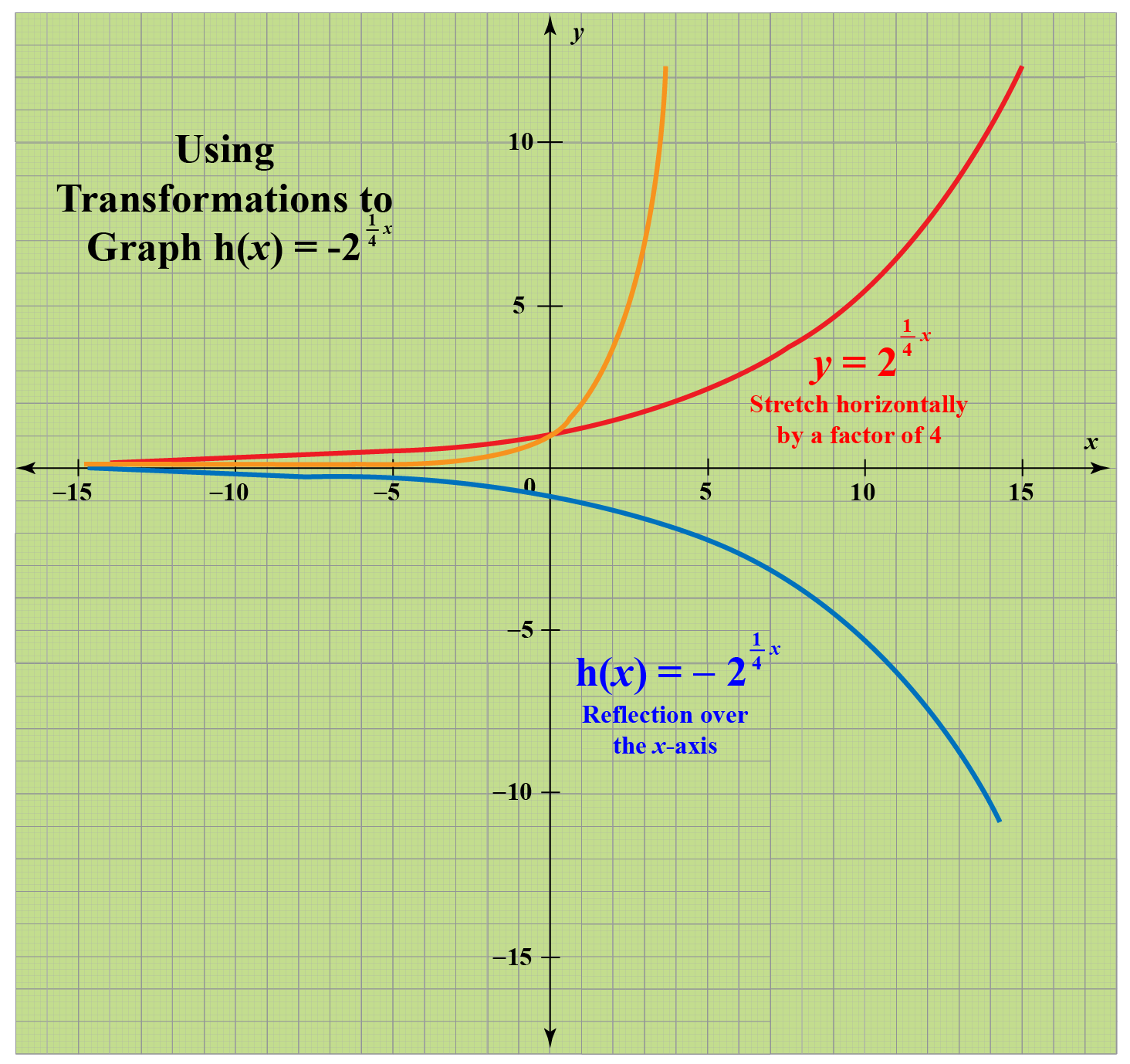This shows the transformation of exponential function $$y=2^x$$.

 So, the graph of the function $$h(x)=-2^{\frac{1}{4}}$$ is shown.
 Example 3

What do the following transformations do to the graph?

i) $$f(x)\rightarrow f(x)-3$$

ii) $$f(x)\rightarrow f(x-3)$$

Solution

$$f(x)\rightarrow f(x)-3$$

The $$y$$-coordinate undergoes the change by 3 units.

Thus, the transformation here is translation 3 units down.

$$f(x)\rightarrow f(x-3)$$

The $$x$$-coordinate undergoes the change of 3 units.

Thus, the transformation here is translation 3 units right.

 $$\therefore$$ i) Translation is 3 units down ii) Translation is 3 units rightThink Tank
1. Can you predict what do the following transformations do to the graph?
(i)  $$f(x)\rightarrow f(x-5)+5$$
(ii)  $$f(x)\rightarrow f(3x+1)-1$$
2. How has the line $$y=x$$ transformed to the line $$y=-x$$?

## Interactive Questions

Here are a few activities for you to practice.

More Important Topics
Numbers
Algebra
Geometry
Measurement
Money
Data
Trigonometry
Calculus
More Important Topics
Numbers
Algebra
Geometry
Measurement
Money
Data
Trigonometry
Calculus
Learn from the best math teachers and top your exams

• Live one on one classroom and doubt clearing
• Practice worksheets in and after class for conceptual clarity
• Personalized curriculum to keep up with school